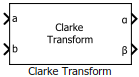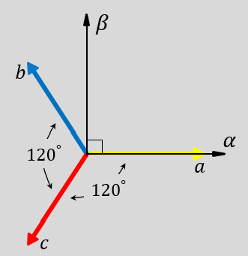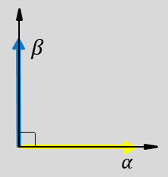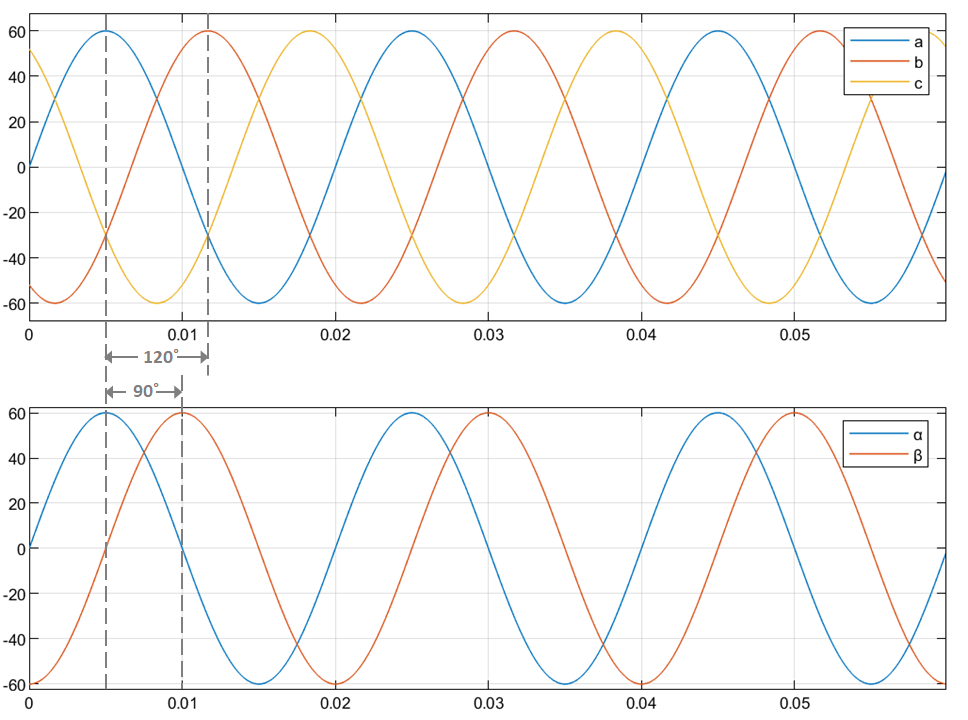# Clarke Transform

Implement ab to αβ transformation

• Library:
• Motor Control Blockset / Controls / Math Transforms

•## Description

The Clarke Transform block computes the Clarke transformation of balanced three-phase components in the abc reference frame and outputs the balanced two-phase orthogonal components in the stationary αβ reference frame.

The block accepts two signals out of the three phases (abc), automatically calculates the third signal, and outputs the corresponding components in the αβ reference frame.

For example, the block accepts a and b input values where the phase-a axis aligns with the α-axis.

• This figure shows the direction of the magnetic axes of the stator windings in the abc reference frame and the stationary αβ reference frame.• This figure shows the equivalent α and β components in the stationary αβ reference frame.• The time-response of the individual components of equivalent balanced abc and αβ systems.### Equations

The following equation describes the Clarke transform computation:

For balanced systems like motors, the zero sequence component calculation is always zero. For example, the currents of the motor can be represented as,

`${i}_{a}+{i}_{b}+{i}_{c}=0$`

Therefore, you can use only two current sensors in three-phase motor drives, where you can calculate the third phase as,

`${i}_{c}=-\left({i}_{a}+{i}_{b}\right)$`

By using these equations, the block implements the Clarke transform as,

where:

• ${f}_{a}$, ${f}_{b}$, and ${f}_{c}$ are the balanced three-phase components in the abc reference frame.

• ${f}_{\alpha }$ and ${f}_{\beta }$ are the balanced two-phase orthogonal components in the stationary αβ reference frame.

• ${f}_{0}$ is the zero component in the stationary αβ reference frame.

## Ports

### Input

expand all

Component of the three-phase system in the abc reference frame.

Data Types: `single` | `double` | `fixed point`

Component of the three-phase system in the abc reference frame.

Data Types: `single` | `double` | `fixed point`

### Output

expand all

Alpha-axis component, α, in the stationary αβ reference frame.

Data Types: `single` | `double` | `fixed point`

Beta-axis component, β, in the stationary αβ reference frame.

Data Types: `single` | `double` | `fixed point`